# Lebesgue constants

The quantitieswhere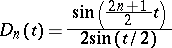is the Dirichlet kernel. The Lebesgue constants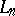for eachequal:

1) the maximum value offor alland all continuous functionssuch thatfor almost-all;

2) the least upper bound offor alland all continuous functionssuch that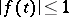;

3) the least upper bound of the integrals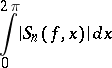for all functionssuch that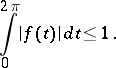Here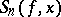is the-th partial sum of the trigonometric Fourier series of the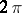-periodic function. The following asymptotic formula is valid: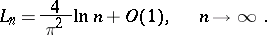In particular,as; this is connected with the divergence of the trigonometric Fourier series of certain continuous functions. In a wider sense the Lebesgue constants are defined for other orthonormal systems (cf. Orthogonal system) as the quantitieswhere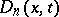is the Dirichlet kernel for the given orthonormal system of functions on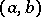; they play an important role in questions of convergence of Fourier series in these systems. The Lebesgue constants were introduced by H. Lebesgue (1909). See also Lebesgue function.